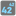• # Gives me error, my local editor says its ok

## Question related to missionIndex Power

My Code:

```def index_power(array, n):
"""
Find Nth power of the element with index N.
"""

if len(array) > n:
return (array[n] ** n)
else:
return (-1)

if __name__ == '__main__':
#These "asserts" using only for self-checking and not necessary for auto-testing
assert index_power([1, 2, 3, 4], 2) == 9, "Square"
assert index_power([1, 3, 10, 100], 3) == 1000000, "Cube"
assert index_power([0, 1], 0) == 1, "Zero power"
assert index_power([1, 2], 3) == -1, "IndexError"
```